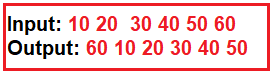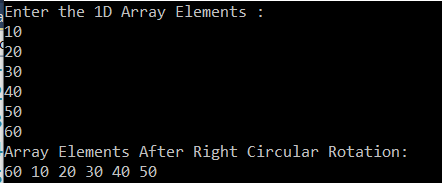# How to Perform Right Circular Rotation of an Array in C#

## How to Perform Right Circular Rotation of an Array in C#

In this article, I am going to discuss How to Perform Right Circular Rotation of an Array in C# with an example. Please read our previous article where we discussed How to Perform Left Circular Rotation of an Array in C# with an example.

##### Program Description:

Here, the user will input a one-dimensional integer array. Then we need to shift each element of the array to its right by one position in a circular fashion. The logic that we need to implement should iterate the loop from 0 t0 Length – 1 and then swap each element with the first element. Please have a look at the following diagram for a better understanding of our requirement.##### Right Circular Rotation of an Array in C#:

In the following example, first we take the input integer array from the user. Then we perform right circular rotation using for loop.

```using System;
namespace LogicalPrograms
{
class Program
{
static void Main(string[] args)
{
int[] oneDimensionalArray = new int;
Console.WriteLine("Enter the 1D Array Elements : ");
for (int i = 0; i<oneDimensionalArray.Length; i++)
{
}

int temp;
for (int j = 0; j < oneDimensionalArray.Length - 1; j++)
{
temp = oneDimensionalArray;
oneDimensionalArray = oneDimensionalArray[j + 1];
oneDimensionalArray[j + 1] = temp;
}

Console.WriteLine("Array Elements After Right Circular Rotation: ");
foreach (int num in oneDimensionalArray)
{
Console.Write(num + " ");
}